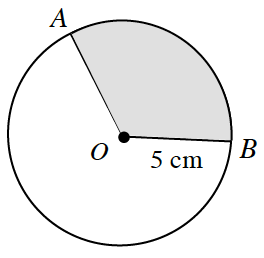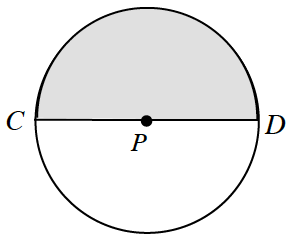### Home > INT2 > Chapter 8 > Lesson 8.4.2 > Problem8-93

8-93.

For each circle below, what are the arc length and the area of the shaded sector? Write your answers in terms of $π$.

1. $m∠AOB = 120^\circ$arc length = (fraction of circle)(circumference of circle)

$\left(\frac{120^\circ}{360^\circ}\right)(2\pi(5))$

$\frac{10\pi}{3}\text{ cm}$

sector area = (fraction of circle)(area of circle)

$\frac{25\pi}{3}\text{ cm}^2$

1. $CD = 6$ inUse the same two methods as part (a). What is the radius of this circle?